# Voltmeter

## Key Stage 3

### Meaning

A voltmeter is a measuring device used to measure the potential difference between two points in a circuit.

Voltmeters are added in parallel to components in a circuit to find the potential difference between two points.
An ideal voltmeter has infinite resistance because otherwise adding an voltmeter to a circuit would change the Potential Difference between two points.
Voltmeters can be analogue with a needle pointing to numbers on a dial or it can be digital with a number display.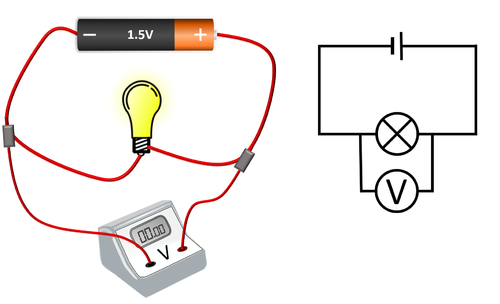A voltmeter placed in parallel with a bulb.

## Key Stage 4

### Meaning

A voltmeter is a measuring device used to measure the potential difference between two points in a circuit.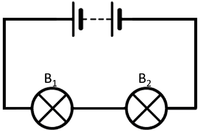To measure the potential difference across bulb 1 the voltmeter must be placed in parallel with bulb 1. To measure the potential difference across bulb 2 the voltmeter must be placed in parallel with bulb 2.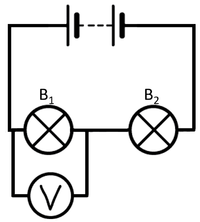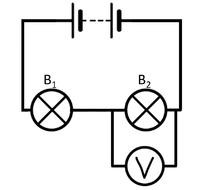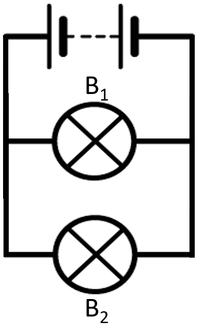To measure the potential difference across bulb 1 the voltmeter must be placed in parallel with bulb 1. To measure the potential difference across bulb 2 the voltmeter must be placed in parallel with bulb 2.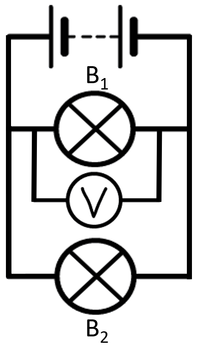In this case both voltmeters will give the same measurement because the components themselves are in parallel.